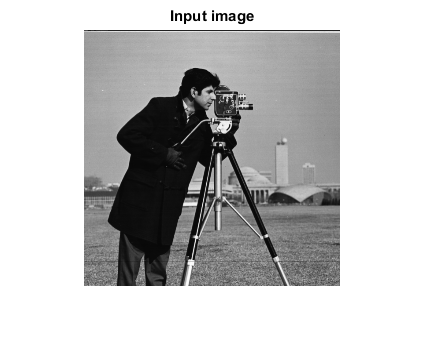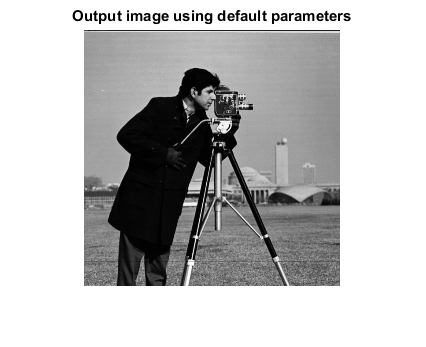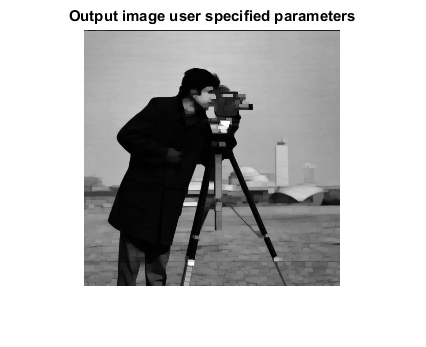# MORPHOPEN_CVIP

morphopen_cvip() - perform morphological opening of a grayscale or color image.

## SYNTAX

`[outImage, struct_el] = morphopen_cvip(inImage, kType, kSize, kArgs)`

Input Parameters include :

• inImage - 1-band or 3-band image of MxN size.
• kType - Kernel or Structure Elements type. kType = 1 ----> disk kType = 2 ----> square kType = 3 ----> rectangle kType = 4 ----> cross (default: 2)
• kSize - Size of Kernel or Structure Elements. Kernel will a square matrix of K*K, where K = kSize. kSize must be positive odd integer (1,3,5, and so on). (default: 3)
• kArgs - Kernel arguments. The number of arguments vary with the kernel type. Any argument must be positive odd integer (1,3,5, and so on) 'disk' kernel, no arguments, i.e. kArgs = [] 'square' kernel, one argument (width) i.e. kArgs = width (default: kSize) 'rectangle' kernel, two arguments (width & height) i.e. kArgs(1) = width kArgs(2) = height (default: [kSize kSize-2]) 'cross' kernel, two arguments (thickness & cross size) i.e. kArgs(1) = thickness kArgs(2) = cross size (default: [1 kSize-2])

Output Parameter includes:

• outImage - Output image of Morphological opening. It is of double class. (Note: Remap or divide by constant C before displaying it, for 8-bit image, C = 255).
• struct_el - Kernel or Structuring element matrix of K*K size.

## DESCRIPTION

The function performs morphological opening of a grayscale image. Morphological Opening is the combination of Erosion followed by Dilation. The user can specify the kernel and its parameters, otherwise the default kernel and kernel parameters are selected.

## REFERENCE

1. Scott E Umbaugh. DIGITAL IMAGE PROCESSING AND ANALYSIS: Applications with MATLAB and CVIPtools, 3rd Edition.

## EXAMPLE

```% Read image

% Call function using default parameters

O1 = morphopen_cvip(I);        %Square kernel with size 3

% Call function using user specified parameters and get two outputs (output image and kernel)

[O2, kernel] = morphopen_cvip(I,3,5,[5 3]); %5*5 rectangle kernel with rectangle width 5 and rectangle height 3

% Display input image

figure;imshow(I);title('Input image');

% Display output image

figure;imshow(O1,[]);title('Output image using default parameters');

figure;imshow(O2,[]);title('Output image user specified parameters');
```## CREDITS

Author: Norsang Lama, June 2017